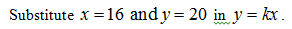find the constant of variation ky varies directly as x. when x is 16, y is 20

Question

find the constant of variation k

y varies directly as x. when x is 16, y is 20

Step 1

Obtain the constant of variation k as follows.

Step 2

It is given that y varies directly as x, which means

Step 3...

Want to see the full answer?

See Solution

Want to see this answer and more?

Our solutions are written by experts, many with advanced degrees, and available 24/7

See Solution
Tagged in

Equations and In-equations